#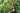While taking data structures and algorithms course on Udemy, I was trying to implement what I just learned onto my Chrome extension project, because the main data of the Chrome extension was stored in an array inefficiently. However, I didn't know what is the best structure, and how I can make changes to the situation.

In this series of articles, we discuss implementations, the pros, and cons of each data structure so we can understand its features, and find out which is the best for the main data.

Here is the main data in an array as an example:

``````// Result of console.log(main-data)
(4)[{...}, {...}, {...}, {...}]
0: {category: "cat1", id: "4", meaning: "information of the vocabulary.", tag: ["tag1", "tag2"], word: "Example Vocab 1"}
1: {category: "cat3", id: "3", meaning: "Hello World", tag: ["tag1", "tag4"], word: "Example Vocab 2"}
2: {category: "cat2", id: "2", meaning: "This is new vocabulary.", tag: ["tag4"], word: "Example"}
3: {category: "cat4", id: "1", meaning: "You can write anything.", tag: ["tag2", "tag4", "tag5"], word: "Sample"}``````

This takes time complexity of O(n) if we wanted to edit/delete each data.

Today we are going to discuss binary search tree and if we can implement it for the main data.

## What is binary search tree look like?

As there is 'tree' in its name, the binary search tree looks like a tree if we visualize it.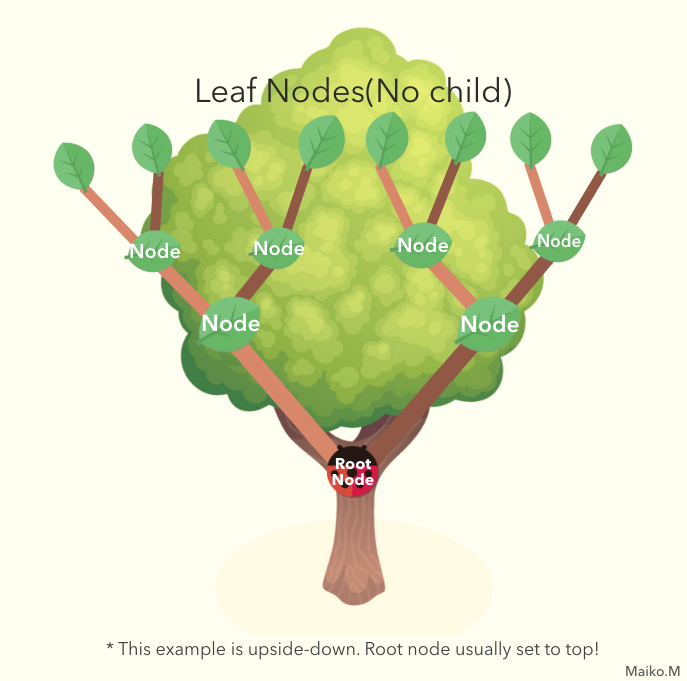Tree is one big group of data structure type and there are many categories within, such as binary trees, Heaps, etc. Each of trees has its own features but they are all nonlinear data structures, not likely arrays, linked lists, stacks and queues which are linear data structures.

Binary search tree is a special case of binary trees that each node can hold 0 to 2 children, but not more than 2. And on top of that, they are sorted in a special order.

Like linked lists, each node can point out their children. As the rule, left pointer can only point out a node that is smaller than the parent node, and right pointer can only point out a node that is larger than the parent.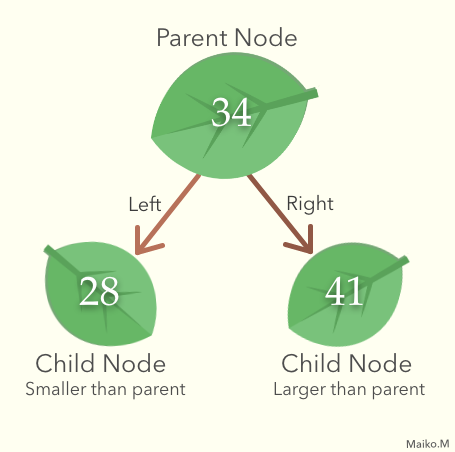These features make binary search tree good at searching. For instance, when you want to find a node 23, you can start from the root node, and if it's not 23 and larger than 23, you only need to search left side of the root.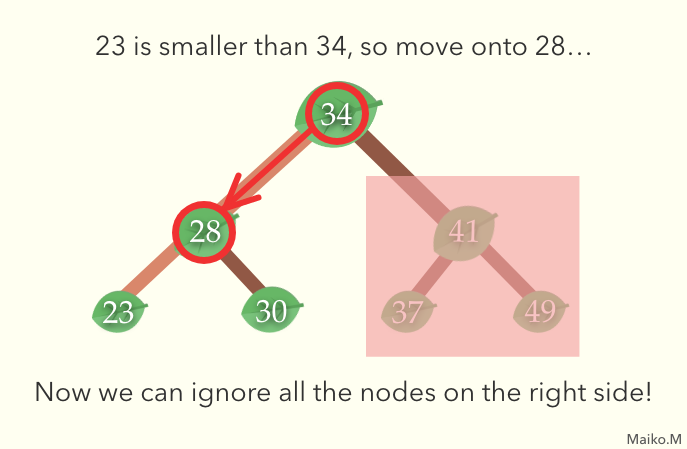## Basic Implementation

Firstly, we define Node and BinarySearchTree. Node has properties of 2 children, and they are defined as left and right.

``````class Node {
constructor(val) {
// store value into val property
this.val = val;
// initialize left child property empty
this.left = null;
// initialize right child property empty
this.right = null;
}
}``````

To define a binary search tree itself, we only need to define root property.

``````class BinarySearchTree {
constructor(){
this.root = null;
}
}``````

### Searching

Searching only cost time complexity of O(log n) because every iteration, you can get rid of half of the nodes in one go. In other words, even you have a double amount of nodes in the tree, you only need to add one more iteration.

``````find(val) {
// define the root node as target
let target = this.root,
// Set found flag as false, and while loop runs when it is false
let found = false;
// Return false if nothing in the tree
if (target === null) return false;
// run while loop when target exists and also 4e2flag is false
while (target && !found) {
if (val < target.val) {
// if the value we are looking for is smaller than the target value, point left child out as target
target = target.left;
} else if (val > target.val) {
// if the value we are looking for is larger than the target value, point right child out as target
target = target.right;
} else if (val === target.val) {
// if the value and the value of the target match, set found flag true
found = true;
}
}
return found;
}``````

### Insertion

Inserting also takes O(log n) with the same reason as searching.

``````insert(val) {
// Create a node
const node = new Node(val);
if(this.root === null) {
// if the tree is empty, append the node as root
this.root = node;
return this;
} else {
// otherwise set root node as target
let target = this.root;
while (true) {
// if same node exists in the tree, return undefined
if (val === target.val) return undefined;
// Case 1: when the node we want to insert is greater than target
if (node.val > target.val) {
if (target.right === null) {
// if the target's right child is empty, set the node in there
target.right = node;
return this;
} else {
// if there is no room at the right of target, set target.right as target
target = target.right;
}
}
// Case 2: when the node we want to insert is lesser than target
if (node.val < target.val) {
if (target.left === null) {
// if the target's left child is empty, set the node in there
target.left = node;
return this;
} else {
// if there is no room at the left of target, set target.left as target
target = target.left;
}
}
}
}
}``````

### Deletion

To delete a node, we need to consider three situations and append different functions to each scenario.

1. When deleting a leaf node Set the parent node's pointer to the leaf node as null

2. When deleting a node with one child Set the parent node's pointer to the deleting node's child node

3. When deleting a node with two children Find the smallest leaf node in the right side of the parent node, then overwrite deleting node with the smallest leaf, and delete the smallest leaf node.

``````delete(val) {
const deleteNode = (node, val) => {
if (!node) return undefined;
if (node.val === val) {
// Case1: When deleting a leaf node
if (node.left === null && node.right === null) {
return null;
}
// Case2: When deleting a node with one child
else if (node.left === null) {
return node.right;
}
else if (node.right === null) {
return node.left;
}
// Case3: When deleting a node with two children
else {
let replacement = node.right;
while(replacement.left !== null) {
replacement = replacement.left;
}
node.val = replacement.val;
node.right = deleteNode(node.right, replacement.val);
return node;
}
} else if (val < node.val) {
// if the target value is larger than the value you are looking for,
//move onto left child
node.left = deleteNode(node.left, val);
return node;
} else {
// if the target value is smaller than the value you are looking for,
//move onto right child
node.right = deleteNode(node.right, val);
return node;
}
}
this.root = deleteNode(this.root, val);
}``````

## Conclusion: Is Binary Search Tree the best choice?

As searching/insertion/deletion take O(log n) complexity, I thought it can be the best choice to implement to my Chrome Extension, however, there are situations to take O(n) for each method. Unfortunately, that could be the case for the project.

With a binary search tree, there is a case to be unbalanced depends on the situation. for example, if the smallest node is appended to the root node, the rest of the node only stored on the right side.

If sorted data was inserted one by one? It would actually be a linked list.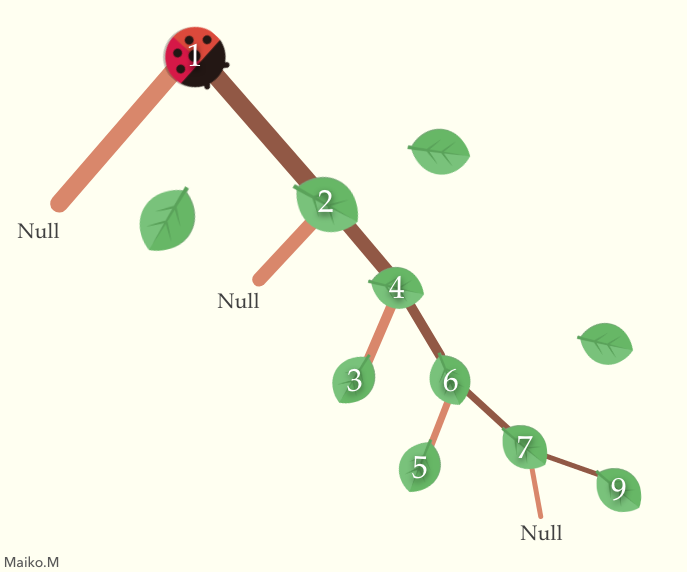Therefore a binary search tree should be implemented with another method to keep the tree balanced, otherwise we may not be able to use the full potential.

I'm going to move forward to see if there's a better structure for my Chrome Extension project, but I'm going to keep binary search tree as one of the options.